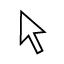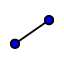Incenter - 2019

Topic:
Geometry

Follow the steps below to construct the incenter on the triangle given above.

Step 1: Useto construct the angle bisectors of angles A, B and C. Step 2: Useto add a point where the three angle bisectors intersect. Step 3: Useto label the point where the angle bisectors intersect.

The point where all three angle bisectors intersect is called the incenter. 1. No matter how you move the triangle, the incenter is always inside the triangle. Useto adjust the triangle. What do you notice about vertex A if it is very close to the incenter?

Check all that apply

2. Useto adjust the triangle so that one vertex is definitely farther from the incenter than the other vertices. What do you notice about the angle measure of that vertex in relation to the other vertices?

Check all that apply

Useto draw the segment from the incenter to point D. Useto draw the segment from the incenter to point E Useto draw the segment from the incenter to point F. 3. These segments show the shortest distance from the incenter to each side of the triangle. Measure the angle between each segment and the triangle side it intersects. What do you notice?

Check all that apply

Incenter Properties

Useto measure the length of each segment from the incenter. Useto drag a vertex of the triangle around. 4. What do you notice about the distance from the incenter to each side of the triangle?

The CircleConstruct a circle that is inside the triangle and touches each side of the triangle once. 5. Why do you think the name incenter was given to the point we are exploring in this activity?

Application for Incenter

6. For which of the following situations, would it make sense to find the incenter?

Check all that apply